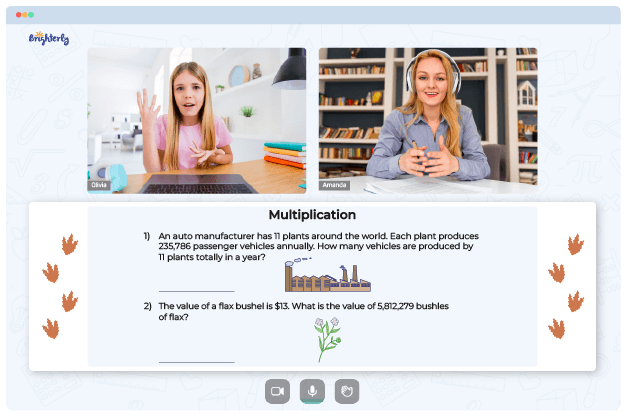# Multi Digit Multiplication Worksheets

One of the great things about multi-digit multiplication is that kids find the operation more challenging than the one with single-digit numbers. The ability to break down large tasks into smaller pieces is a vital skill in many facets of life. And though it may be tricky at first, as soon as a child practices enough, they set the foundation for nailing many other math concepts.

Improvement of your kids’ multiplication skills by solving math problems with multi-digit multiplication worksheets gives them a learning advantage over their peers. The tools contain unique math problems that keep students on their toes while not being too complicated. More teachers are adopting these worksheets in their math lessons, and you should join them.

## Printable Multi-digit Multiplication Worksheets

The set of printable multiplication worksheets includes a variety of exercises in a format you can print. They are the most challenging worksheets for kids but are perfect for students who already know how to multiply a number or two and are looking for something more complicated.

Math for Kids

Is Your Child Struggling With Math?
1:1 Online Math TutoringTeachers can benefit from the fact that they can download the resources online, print them, and share them with students. These features make the resource easy to acquire and dirt cheap.

## The Benefits Of Multi-digit Multiplication Worksheets

Multiple digit multiplication worksheets help children learn and understand the principles of multi-digit multiplication. Worksheets that teach multi-digit multiplication build children’s confidence in math. These multiple digit addition worksheets also increase students’ interest in problem-solving, preparing them for life after school.### Multi Digit Multiplication Worksheets PDF

Multi Digit Multiplication Worksheet### Multi Digit Multiplication Worksheets PDF

Multi-Digit Multiplication Worksheets### Multi Digit Multiplication Worksheets PDF

Multiple Digit Multiplication Worksheets### Multi Digit Multiplication Worksheets PDF

Multiplying Multi Digit Numbers Worksheets

Even though these worksheets are more advanced than single-digit multiplication ones, they still follow a progressive approach in the question format. Easy sheets help a child warm up before the complicated tasks. Once children learn the fundamental solving process and pattern, other math tasks — including those unrelated to multiplication — become easier.

The sheets are also great as a type of formative assessments and task assignments. Learning math with a multi digit multiplication worksheet provides students with a quick reference point in case they forget a formula and want to recover or remember it.

### More Multiplication Worksheets

Struggling with Multiplication?• Does your child struggle to master the basics of multiplication?
• Try lessons with an online tutor.

Is your child having trouble understanding multiplication? An online tutor could provide the necessary help.